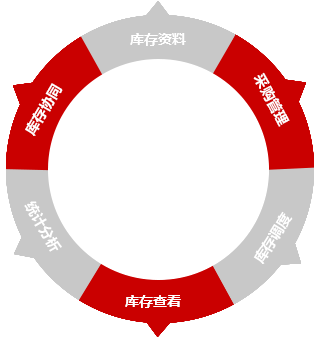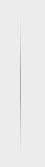STOCK SOLUTION• 核心功能
• 多仓库
• 多部门
• 多员工
• 多电商
• 多店商
• 多系统
• 电脑端
• 移动端
• 微信端
• 应用工具
• 条形码
• 二维码
• 序列号
• 批号批次
• 保质期
• 扫描枪
• 采集器
• 盘点机
• 打印机

• 全域仓库管理
• 库存实时更新
• 采购全程可视
• 仓库自由调度
• 仓库独立核算
• 仓储效率提升
• 多仓库
• 多区域
• 多部门
• 多人员
• 多平台
• 多店铺
• 多业务
• 多终端

• 线上库存
• 线下库存
• 无缝对接
• 自动流转
• 数据同步
• 实时更新
• 库存精准
• 智能查询

• 供应商
• 询价
• 预购
• 采购
• 审批
• 到货
• 质检
• 入库

• 直接出入库
• 调拨出入库
• 盘点出入库
• 采购入库
• 退货入库
• 销售出库
• 借货出库
• 自由调拨

• 多仓库
• 多单位
• 多分类
• 多库位
• 各区域
• 各公司
• 各部门
• 各库管

• 条形码
• 二维码
• 扫描枪
• 序列号
• 批号批次
• 保质期
• 采集器
• 盘点机

400-650-8060

+ 智邦一体化ERP
+ 集团及分公司

+ 关于我们
+ 联系我们

400热线：400-650-8060

+ 反馈中心扫一扫关注

400-650-8060

X

X